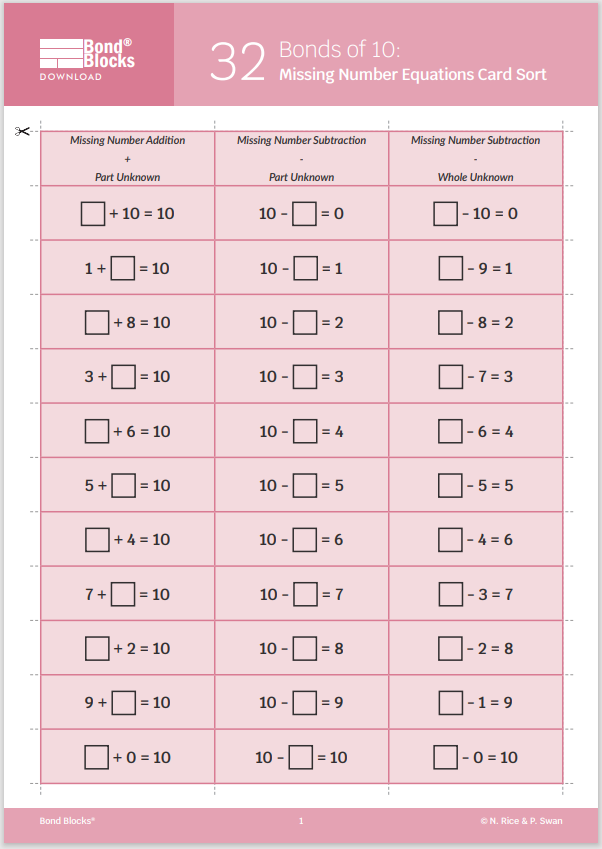## 33 Representing Addition and Subtraction

Bonds of 10: Thinkboard

### Mathematics

Develop the concept of addition and subtraction using Bond Blocks, diagrams, an equation, pictures and word story.

Develop fluency recalling a difficult to remember Bond of 10.

### Language

• bond
• part-part-whole
• subtraction## Differentiation

### A little easier

The teacher completes one thinkboard for a selected two-part bond of ten. Students use this as a model, repeating the teacher’s thinkboard, applying it to a different two-part bond of ten.

When modelling word problems refer to the “Solving Word Problem” in regards to modelling a variety of problem types. Students typically find active problems easier than static ones and Part-Part-Whole problems easier than comparison problems. Ensure students are exposed to a range of problem types for both addition and subtraction.

##### Build a repertoire

Encourage students to share their thinkboards with each other so as students can build a repertoire of word stories and representations of the operation. Students can then adapt their friends’ examples by changing the nouns (eg cars for dogs) or the numbers (a different bond of 10). Changing the nouns is easier than changing the numbers.

##### Act it out

Assist students to understand the problem by instructing them them to act out of the equation before representing it with pictures and words. Students can act out the problem with:

• Actual objects (for example, toy cars) that feature in their problem.
• Objects such as blocks that represent the actual object. This is more difficult than using actual objects.

### A little harder

##### Create word questions

Instruct students to create:

• A word question on a blank piece of paper, for another student to answer, and
• A matching answer page for the question they have written. Use the Bonds of 10: Representing Addition Thinkboard activity board as a template for the answer page.

Students can be encouraged to create word questions where

• There are more than two parts.
• The unknown is not in the answer position. Support students to create these questions by clicking to download the Bonds of 10: Missing Number Equations Card Sort from the previous activity. These cards have equations written where the missing number is not in the answer position. Students can select a card, represent the answer on the Thinkboard and then use this to write a matching word question.### Progression

In the next chapter of activities students focus on remaining bonds for the wholes 6, 7, 8, and 9 that have not yet been covered. The sequence of activities in this chapter is similar to past chapters: moving from identify bonds, to developing fluency, calculating, solving missing number equations and word problems. Go to

##### Activity 34

Bonds of 6, 7, 8, 9: Bonds, Building a Wall